edraw-max-horizontal
Guide
What's New
TRY IT FREE

Explain Algorithm and Flowchart with Examples

> Flowchart Examples > Explain Algorithm and Flowchart with Examples
Algorithm and flowchart are two types of tools to explain the process of a program. This page extends the differences between an algorithm and a flowchart, and how to create a flowchart to explain an algorithm in a visual way.

Algorithms and flowcharts are two different tools used for creating new programs, especially in computer programming. An algorithm is a step-by-step analysis of the process, while a flowchart explains the steps of a program in a graphical way.

Definition of Algorithm

To write a logical step-by-step method to solve the problem is called algorithm, in other words, an algorithm is a procedure for solving problems. In order to solve a mathematical or computer problem, this is the first step of the procedure. An algorithm includes calculations, reasoning and data processing. Algorithms can be presented by natural languages, pseudo code and flowcharts, etc.

Definition of Flowchart

A flowchart is the graphical or pictorial representation of an algorithm with the help of different symbols, shapes and arrows in order to demonstrate a process or a program. With algorithms, we can easily understand a program. The main purpose of a flowchart is to analyze different processes. Several standard graphics are applied in a flowchart:

• Terminal Box - Start / End
•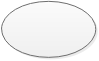• Input / Output
•• Process / Instruction
•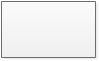• Decision
•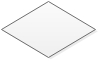• Connector / Arrow
•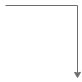The graphics above represent different part of a flowchart. The process in a flowchart can be expressed through boxes and arrows with different sizes and colors. In a flowchart, we can easily highlight a certain element and the relationships between each part.

How to Use Flowcharts to Represent Algorithms

Now that we have the definitions of algorithm and flowchart, how do we use a flowchart to represent an algorithm?

Algorithms are mainly used for mathematical and computer programs, whilst flowcharts can be used to describe all sorts of processes: business, educational, personal and of course algorithms. So flowcharts are often used as a program planning tool to visually organize the step-by-step process of a program. Here are some examples:

Example 1: Print 1 to 20:

Algorithm:

Step 1: Initialize X as 0,

Step 2: Increment X by 1,

Step 3: Print X,

Step 4: If X is less than 20 then go back to step 2.

Flowchart: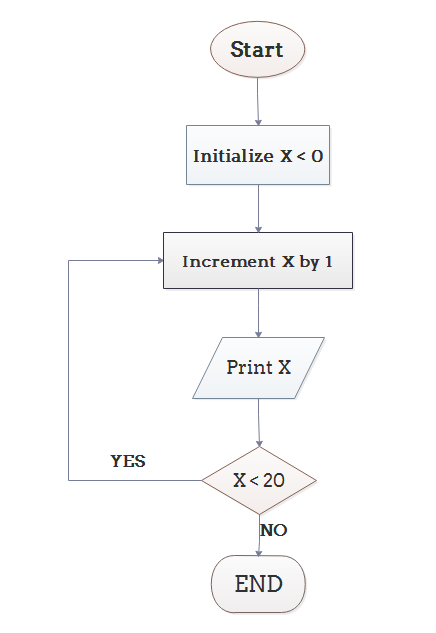Example 2: Convert Temperature from Fahrenheit (℉) to Celsius (℃)

Algorithm:

Step 1: Read temperature in Fahrenheit,

Step 2: Calculate temperature with formula C=5/9*(F-32),

Step 3: Print C,

Flowchart: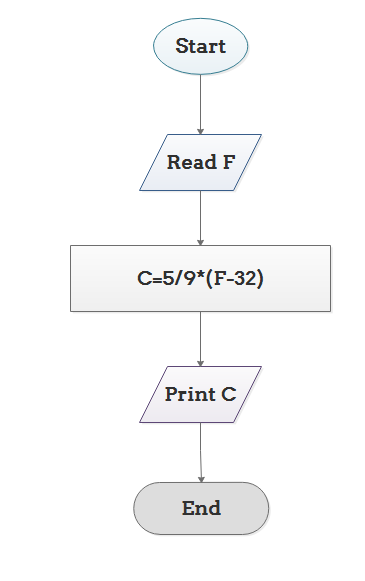Conclusion

From the above we can come to a conclusion that a flowchart is pictorial representation of an algorithm, an algorithm can be expressed and analyzed through a flowchart.

An algorithm shows you every step of reaching the final solution, while a flowchart shows you how to carry out the process by connecting each step. An algorithm uses mainly words to describe the steps while a flowchart uses the help of symbols, shapes and arrows to make the process more logical.